Course Content

# Linear Regression with Python

Linear Regression with Python

## Overfitting

Consider the two regression lines below. Which one is better?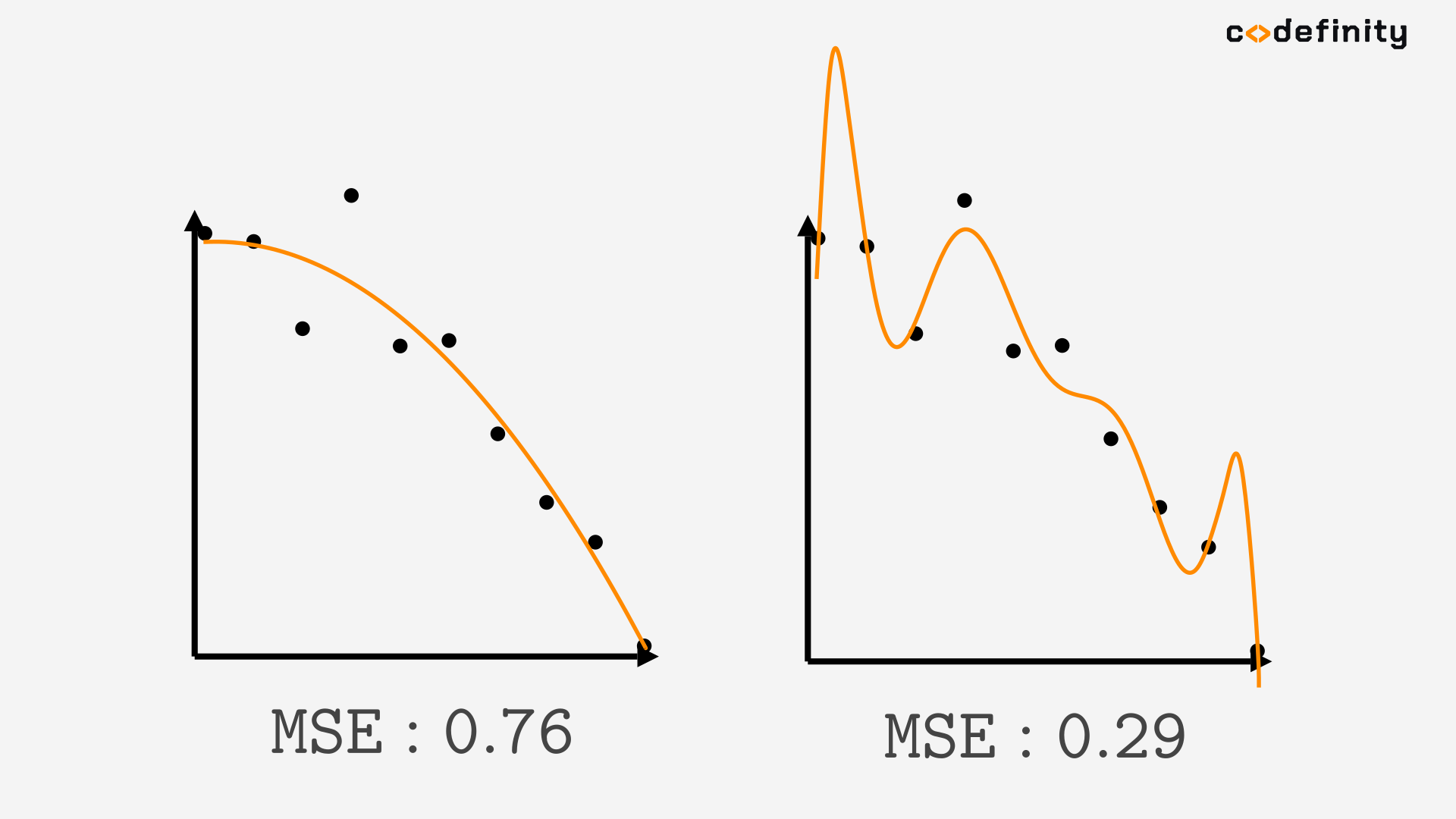Well, the metrics tell us that the second is better. Then let's use it to predict new values! We need the predictions for `X_new = [0.2,0.5,2.7]`. But when we got the actual target values for the `X_new` we just predicted, it turned out that the first model had a much better prediction.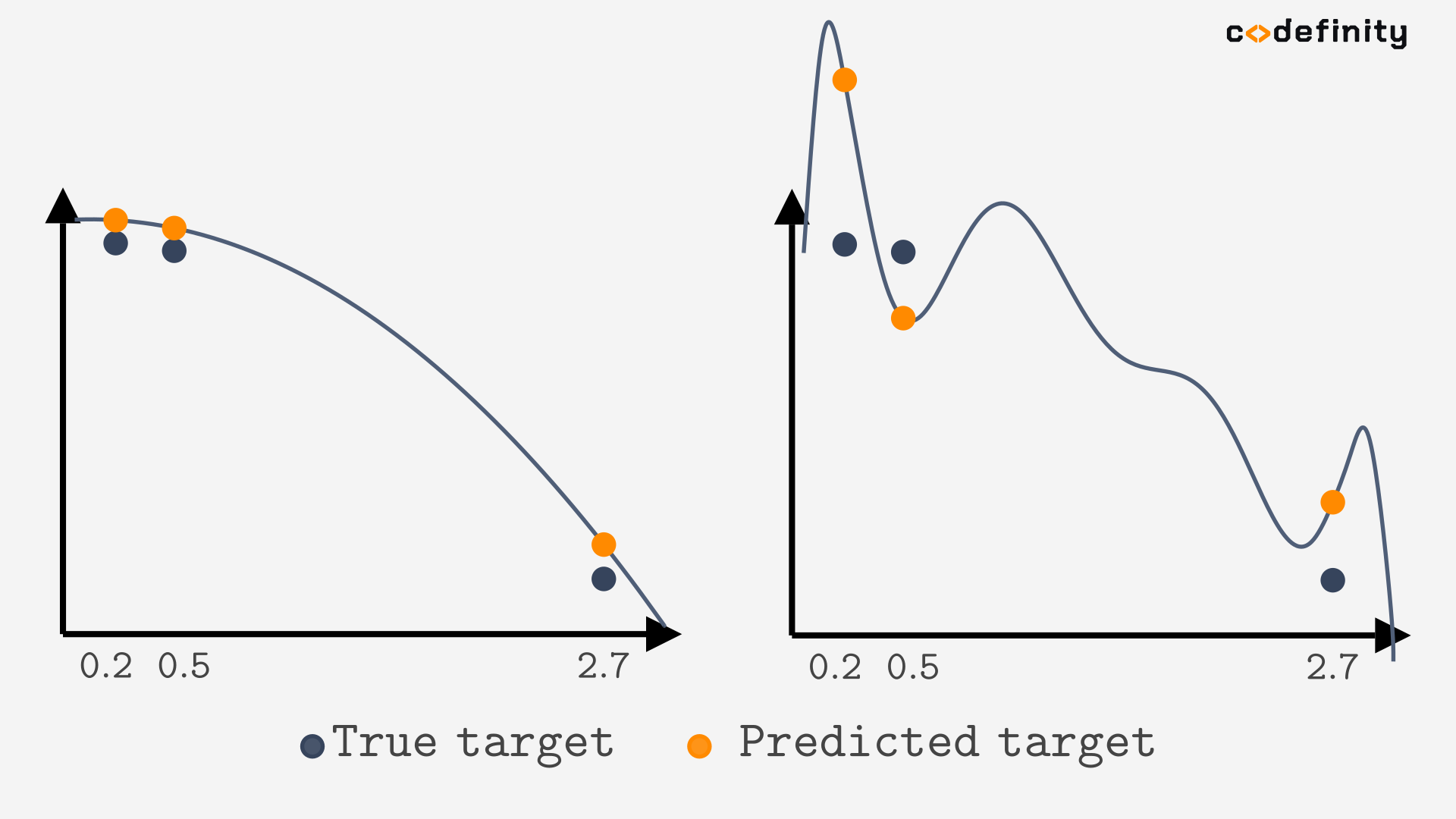That is because the second model overfits the training set. Overfitting is when the built model is too complex so that it can perfectly fit the training data, but it does not predict unseen instances that well.

## Underfitting

There is also one more concept, underfitting. It is when the built model is too simple that it does not even fit the training data well. In that cases, predictions of the unseen instances are wrong too.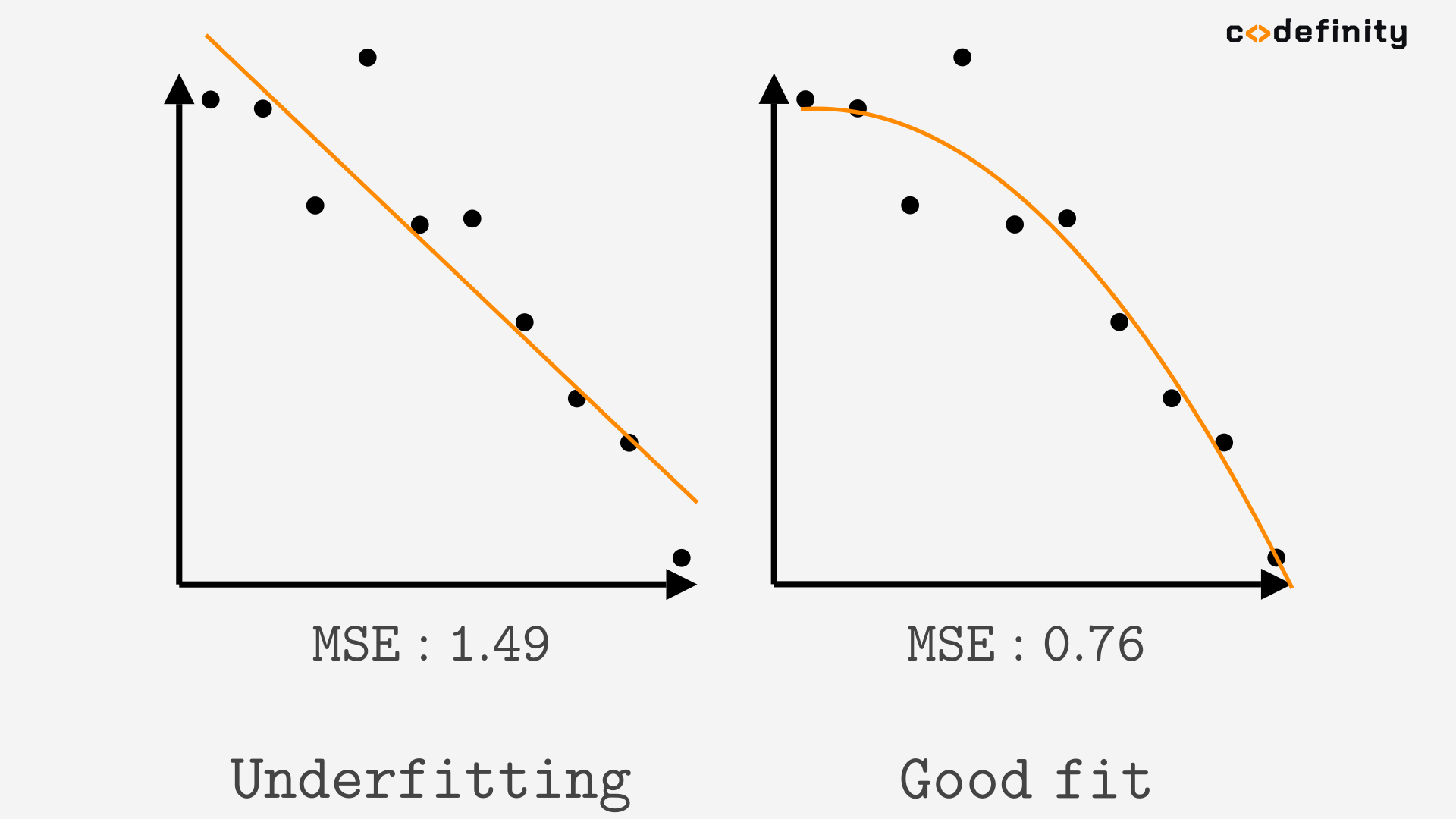So we can try to tell whether the model underfits or overfits visually.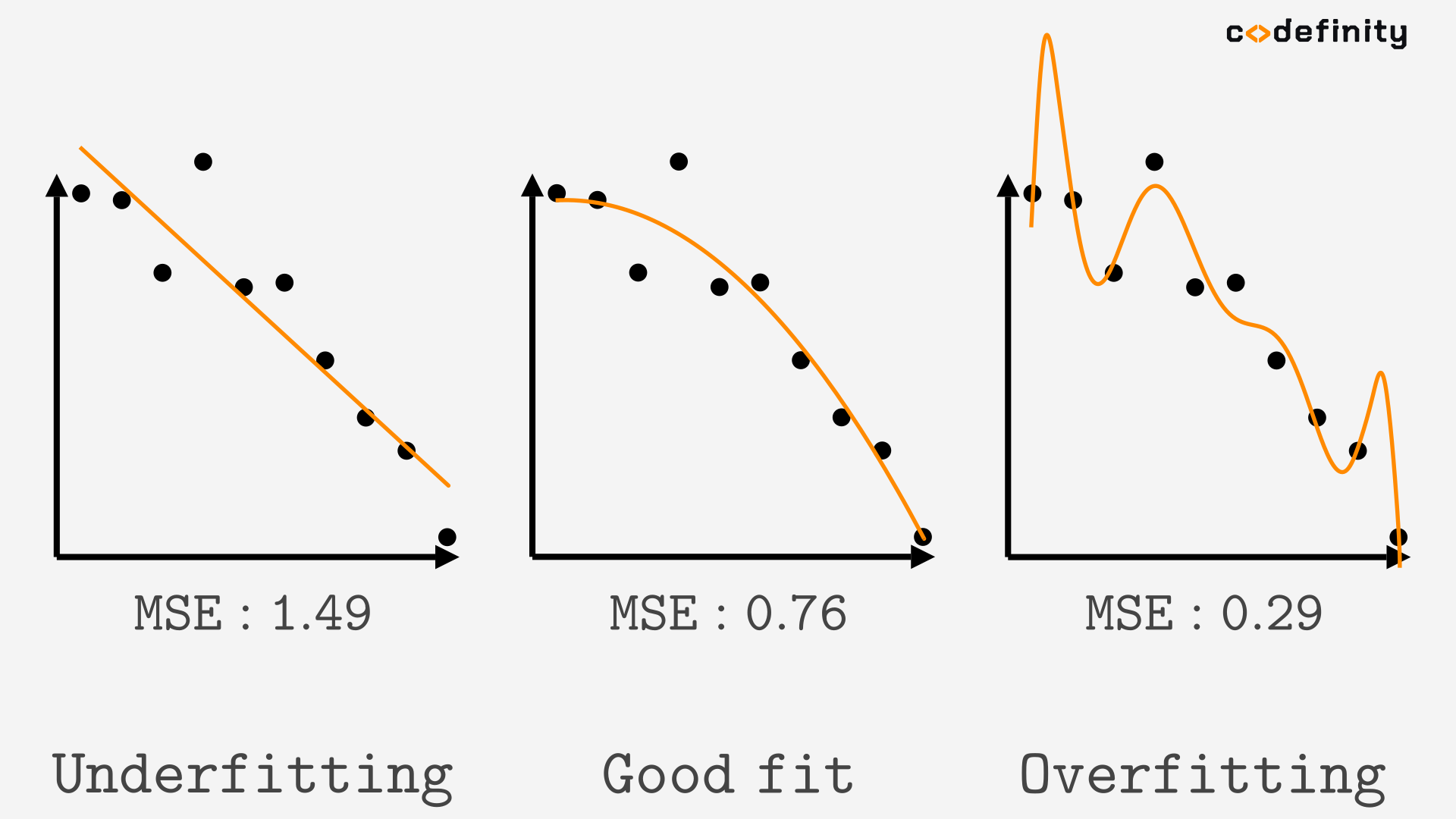We already know that we cannot visualize Multiple Linear Regression with many features. Is there another way to tell whether the model overfits or underfits? It turns out there is.

## Train-test split

We want to know how the model will perform on unseen instances. For that, we need the unseen instances with the true target values. And we only have true target values in the training set. The way to go is splitting the training set into two sets, a training set and a test set.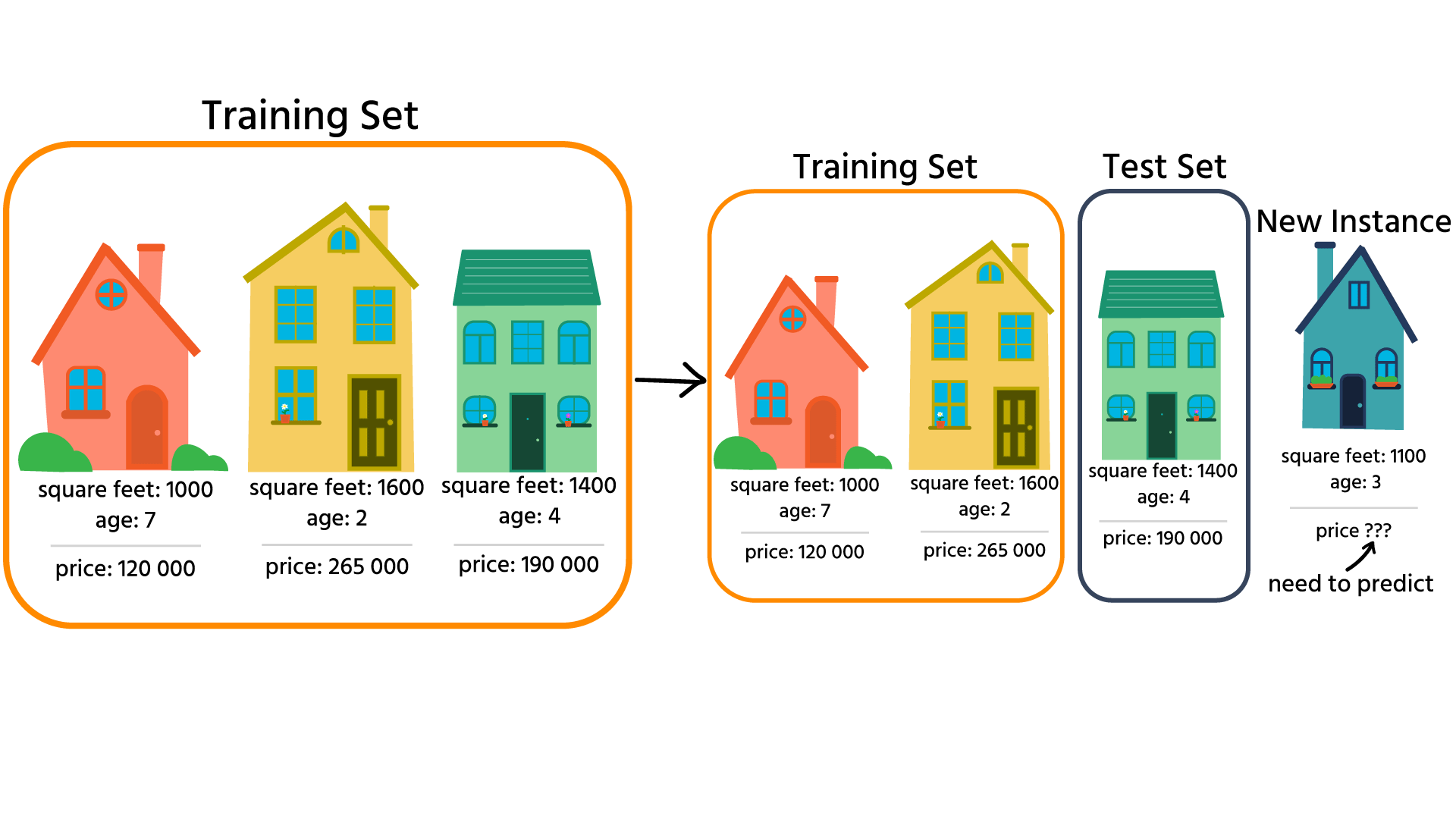Now we can build the model using the training set, calculate the metrics on the training set(seen instances) and then calculate the metrics on the test set(unseen instances).It is essential to split the training set randomly. Usually, you will want to use around 20-30% of your training set for the test set, and the other 70-80% will be left as a training set. Scikit-learn provides a simple function for splitting the set randomly: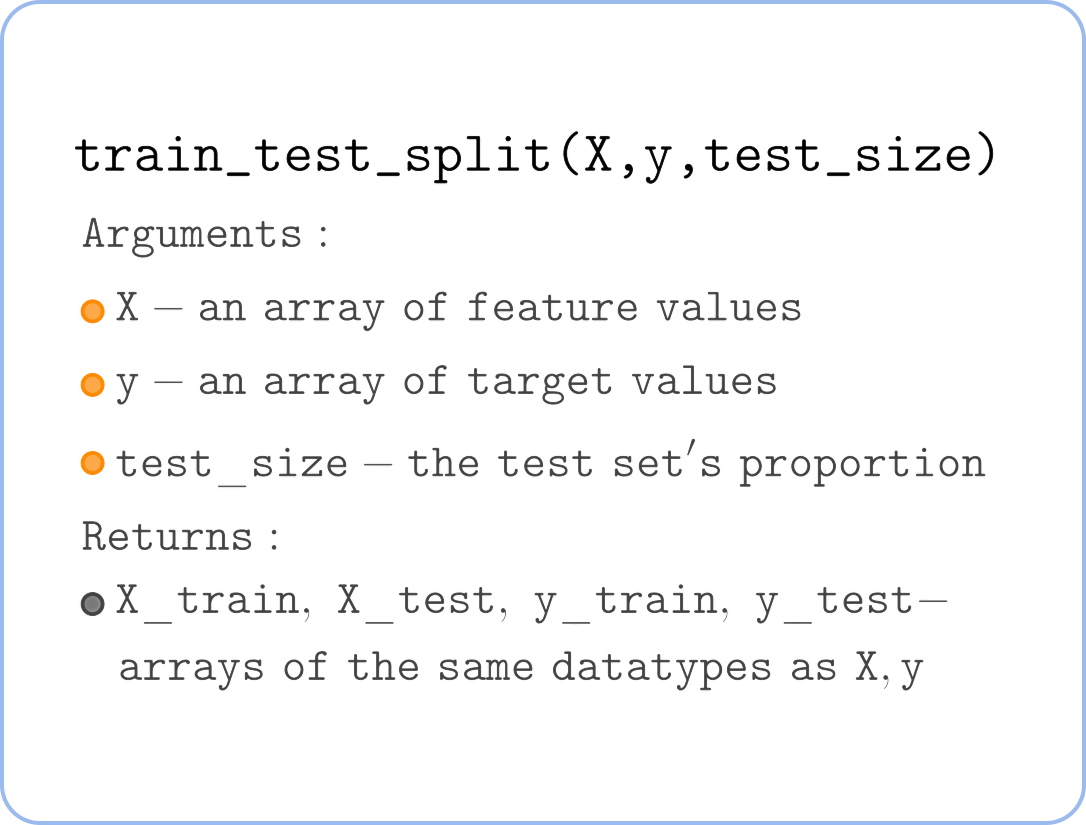For example, to split the training set to 70% training/30% test, you can use the following code:#### Based on MSE scores of the models find out whether they overfit/underfit the training set(dataset is the same).

Model 1: Training set's MSE=0.2, Test set's MSE=0.215
_ _ _
.
Model 2: Training set's MSE=0.14, Test set's MSE=0.42
_ _ _
.
Model 3: Training set's MSE=0.5, Test set's MSE=0.47
_ _ _
.

Click or drag`n`drop items and fill in the blanksUnderfittingOverfittingGood FitEverything was clear?

Section 4. Chapter 2